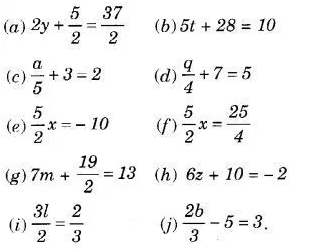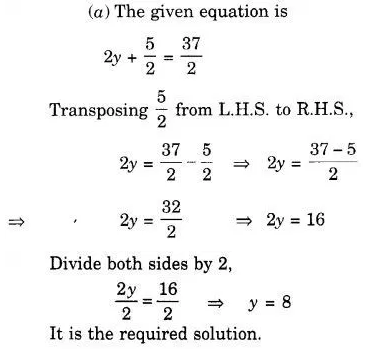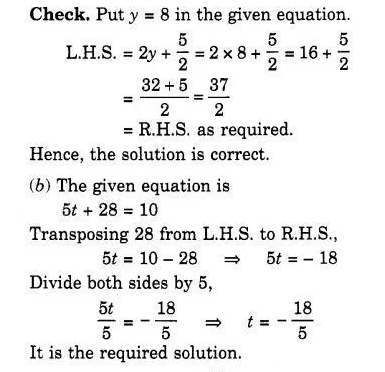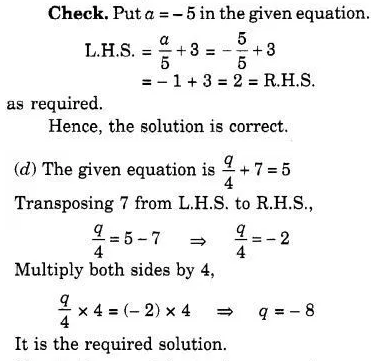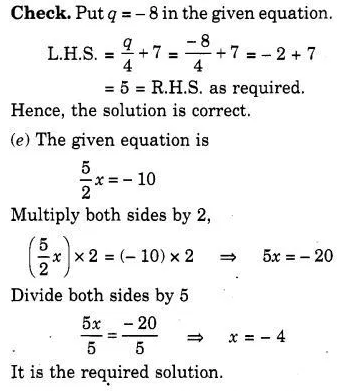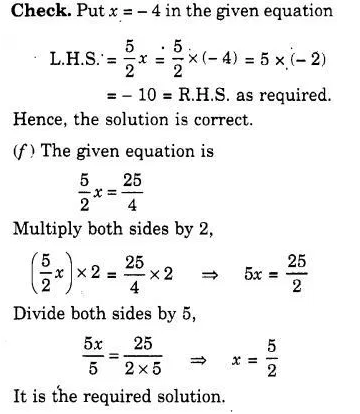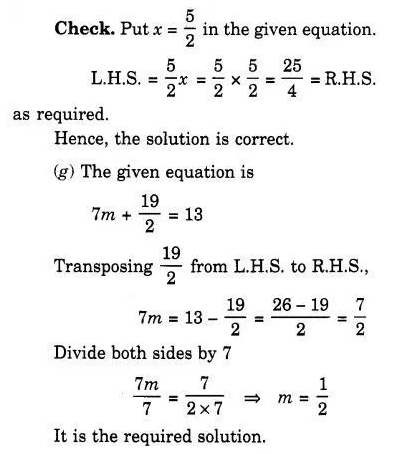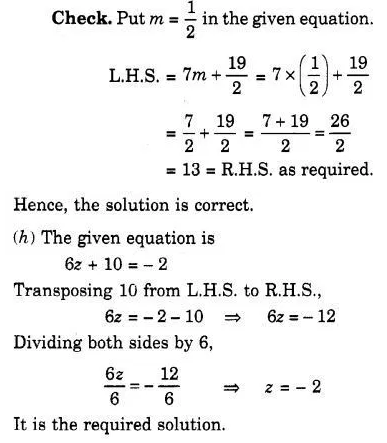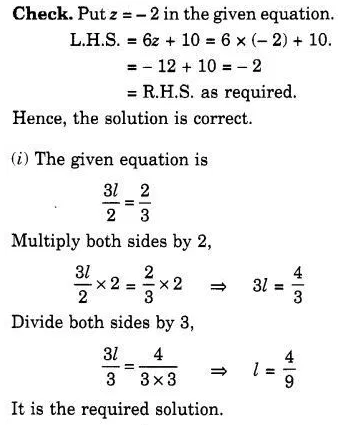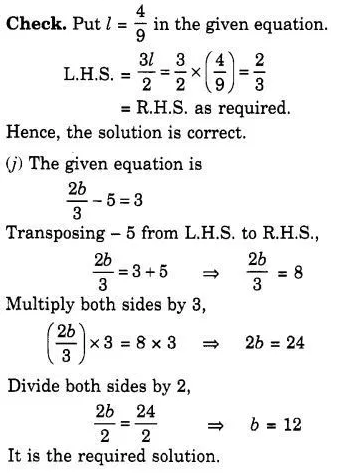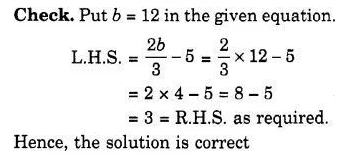# Solve the following equations : (a) 2y + 5/2 = 37/2

Solve the following equations :